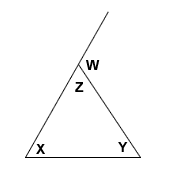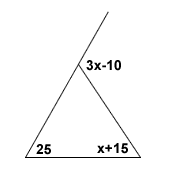# There are angles on the outside, too!

### Definition of an exterior angle

At each vertex of a triangle, an exterior angle of the triangle may be formed by extending ONE SIDE of the triangle. See picture below.### Calculating the Angles

We can use equations to represent the measures of the angles described above. One equation might tell us the sum of the angles of the triangle. For example,

x + y + z = 180

We know this is true, because the sum of the angles inside a triangle is always 180 degrees. What is w? We don't know yet. But, we may observe that the measure of angle w plus the measure of angle z = 180 degrees, because they are a pair of supplementary angles. Notice how Z and W together make a straight line? That's 180 degrees. So, we can make a new equation:

w + z = 180

Then, if we combine the two equations above, we can determine that the measure of angle w = x + y. Here's how to do that:

x + y + z = 180 (this is the first equation)
w + z = 180 (this is the second equation)

Now, rewrite the second equation as z = 180 - w and substitute that for z in the first equation:

x + y + (180 - w) = 180
x + y - w = 0
x + y = w

Interesting. This tells us that the measure of the exterior angle equals the total of the other two interior angles. In fact, there is a theorem called the Exterior Angle Theorem which further explores this relationship:

### Exterior Angle Theorem

The measure of an exterior angle (our w) of a triangle equals to the sum of the measures of the two remote interior angles (our x and y) of the triangle.

Let's try two example problems.

#### Example A:

If the measure of the exterior angle is (3x - 10) degrees, and the measure of the two remote interior angles are 25 degrees and (x + 15) degrees, find x.To solve, we use the fact that W = X + Y. Note that here I'm referring to the angles W, X, and Y as shown in the first image of this lesson. Their names are not important. What is important is that an exterior angle equals the sum of the remote interior angles.

We equate and solve for x.

exterior angle = interior angle + other interior angle

$$(3x - 10) = (25) + (x + 15)$$ $$3x - 10 = x + 40$$ $$3x = x + 50$$ $$2x = 50$$ $$x = 25$$

Remember that "x" is not the answer here. We need the angles themselves, which are calculated as (3x-10), 25, and (x+15). The angles, then, are 65, 25, and 40 degrees.

#### Example B

The exterior angle given is 110 degrees. Two remote interior angles measure 50 and (2x + 30). Find x.

Remember: exterior = sum of remote interior angles

We're given the exterior angle (110). We equate 110 to (2x + 30) + 50 and solve for x.

$$110 = 2x + 30 + 50$$ $$110 = 2x + 80$$ $$30 = 2x$$ $$15 = x$$

A lesson provided by Mr. Feliz